# can you solve these problems with derivative (local max,min, concavity etc.) also, can you find its extrema and show the graph? 2 2-4 tį 2 2-4 tį

can you solve these problems with derivative (local max,min, concavity etc.) also, can you find its extrema and show the graph?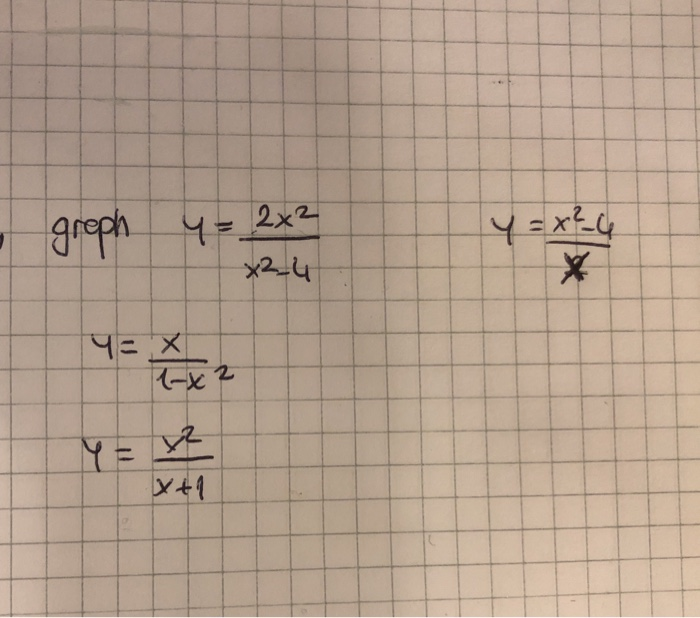2 2-4 tį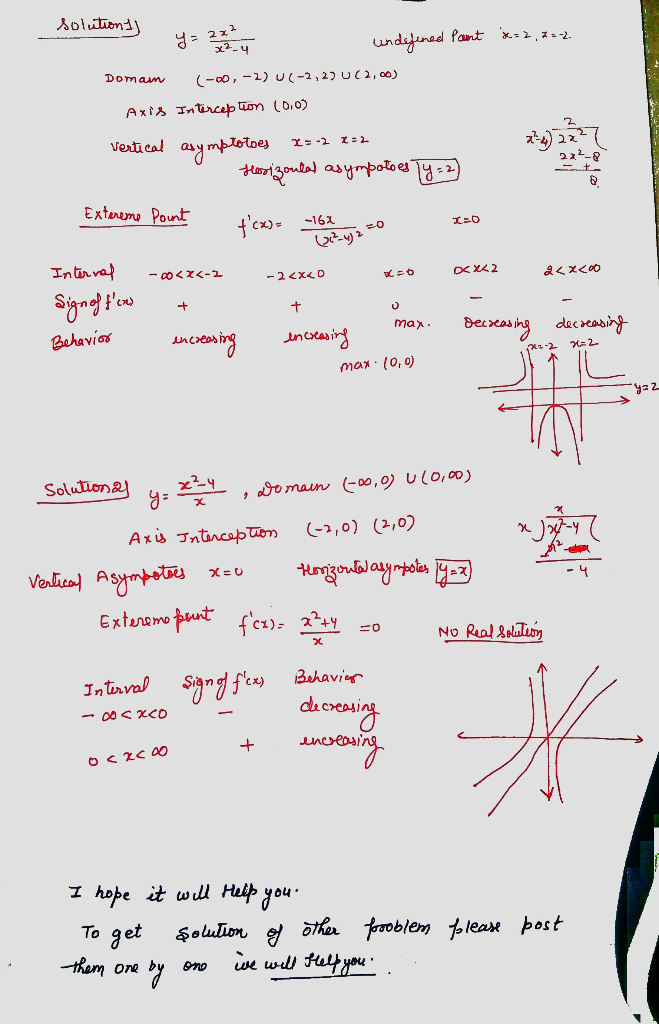##### Add Answer of: can you solve these problems with derivative (local max,min, concavity etc.) also, can you find its extrema and show the graph? 2 2-4 tį 2 2-4 tį
Similar Homework Help Questions
• ### let g(x)= x^2/x2-4 find domain intercepts asymptotes intervals concavity symmetry local max/min and inflection points

let g(x)= x^2/x2-4 find domain intercepts asymptotes intervals concavity symmetry local max/min and inflection points

• ### 3. Find the equation of the tangent line to the graph of f(x)-1+e 0 4 Graph the following functio...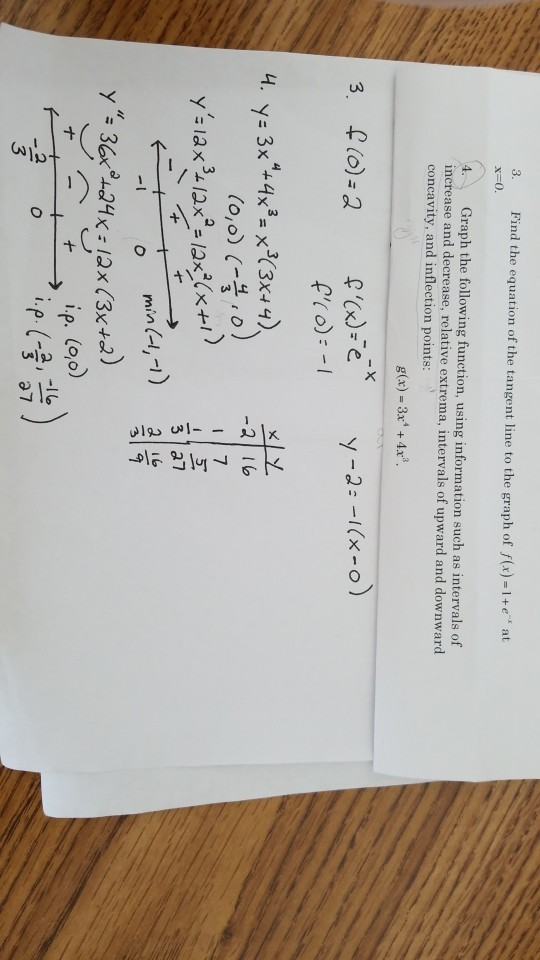Could you label and explain how to get each term? Thank you! 3. Find the equation of the tangent line to the graph of f(x)-1+e 0 4 Graph the following function, using information such as intervals of increase and decrease, relative extrema, intervals of upward and downward concavity, and inflection points: g(x) 3x4 +4.x Pro):-I -2 16 3 a7 al 16 min(-1,-1) y " 30+24K: 12x(3x+2) t ip. (oo) 2 3 3. Find the equation of the tangent line to...

• ### Max 4x1 - 22 + 2.T3 Subiect to: 2x1 + x2 7 Min 4 + 2x2 - T3 Subject to: x1 + 2x2-6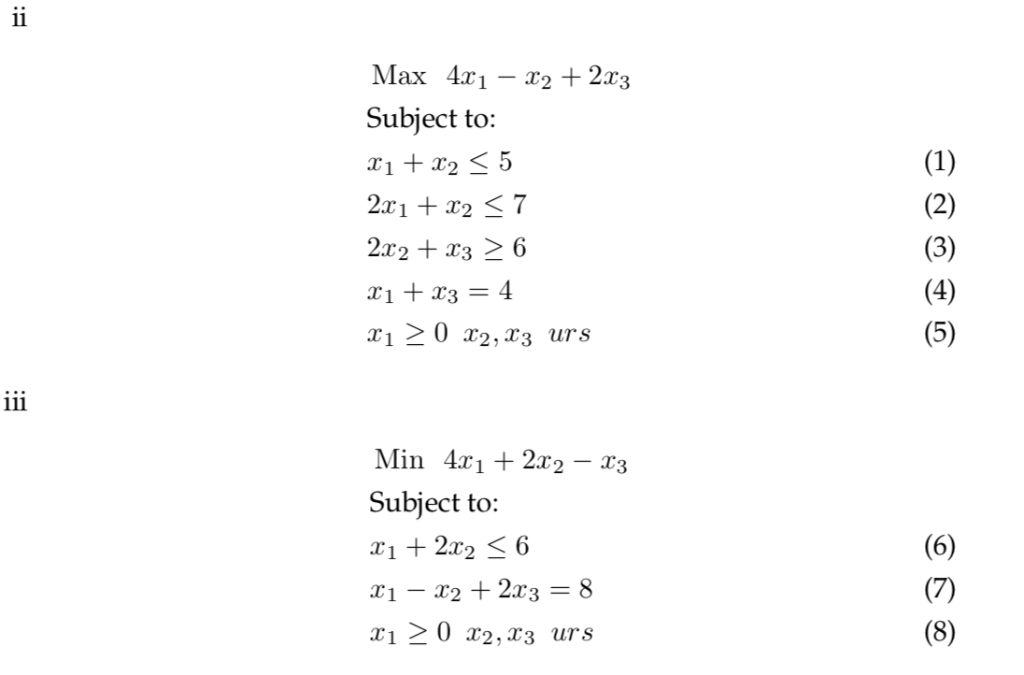Determine the Dual of the following Linear Programming Problems Max 4x1 - 22 + 2.T3 Subiect to: 2x1 + x2 7 Min 4 + 2x2 - T3 Subject to: x1 + 2x2-6 Max 4x1 - 22 + 2.T3 Subiect to: 2x1 + x2 7 Min 4 + 2x2 - T3 Subject to: x1 + 2x2-6

• ### (a) A function / has first derivative f'(z) = and second derivative 3) f"(x) It is also known that the function...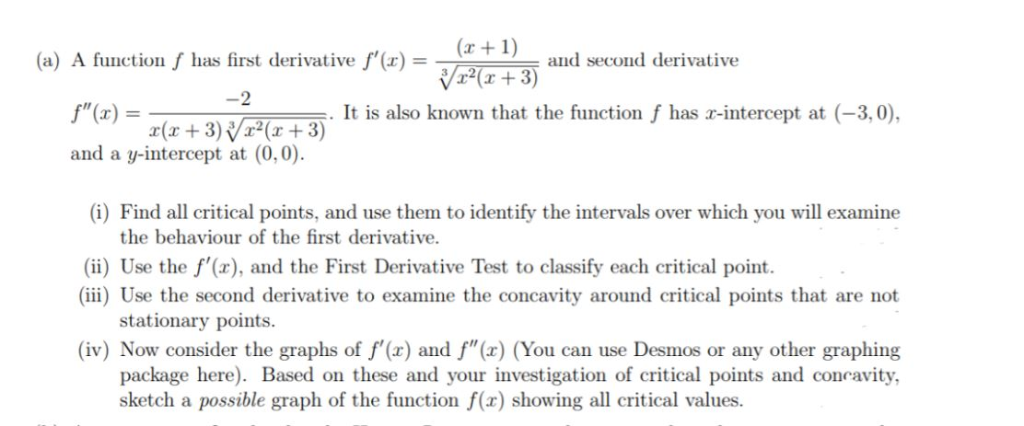(a) A function / has first derivative f'(z) = and second derivative 3) f"(x) It is also known that the function f has r-intercept at (-3,0), and a y-intercept at (0,0) (i) Find all critical points, and use them to identify the intervals over which you will examine the behaviour of the first derivative ii) Use the f'(), and the First Derivative Test to classify each critical point. (ii) Use the second derivative to examine the concavity around critical points...

• ### 38-40. Assume that the fol lowing is the entire graph of fx). 38 Which of the following are local maximums for fx)? A) (-9/4, 3) E) (2, 2) 9 Which of the following are global extrema for f(x)? A) (-9...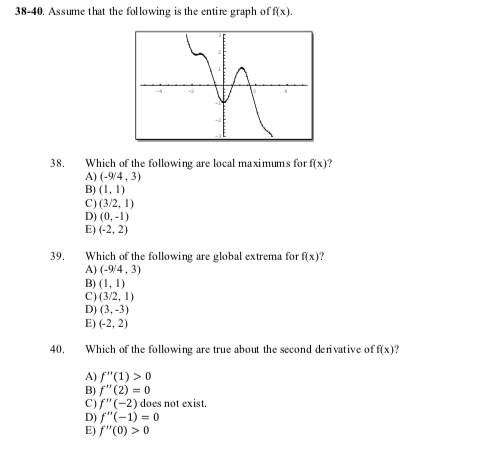38-40. Assume that the fol lowing is the entire graph of fx). 38 Which of the following are local maximums for fx)? A) (-9/4, 3) E) (2, 2) 9 Which of the following are global extrema for f(x)? A) (-9/4,3 40. Which of the following are true about the second derivative of f(x)? B)f (2) 0 c)f" (-2) does not exist. D) f"(-1) = 0 E "CO)> 38-40. Assume that the fol lowing is the entire graph of fx). 38...

• ### 2 012 (6 pts). Find the critical points of y and use the second derivative xe 2 1+y2 test to classify these points as saddle, local minimum and local maximum points. 2 012 (6 pts). Find the c...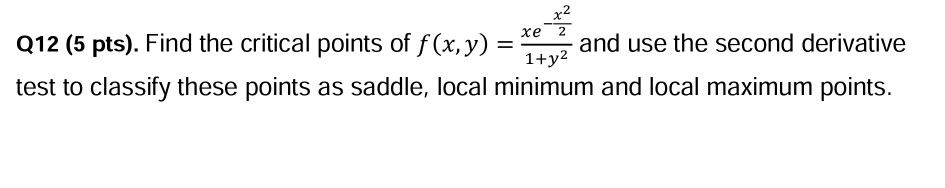2 012 (6 pts). Find the critical points of y and use the second derivative xe 2 1+y2 test to classify these points as saddle, local minimum and local maximum points. 2 012 (6 pts). Find the critical points of y and use the second derivative xe 2 1+y2 test to classify these points as saddle, local minimum and local maximum points.

• ### 4. (a) A function f has first derivative f'(x) and second derivative 2 f" (x) It is also known that the functio...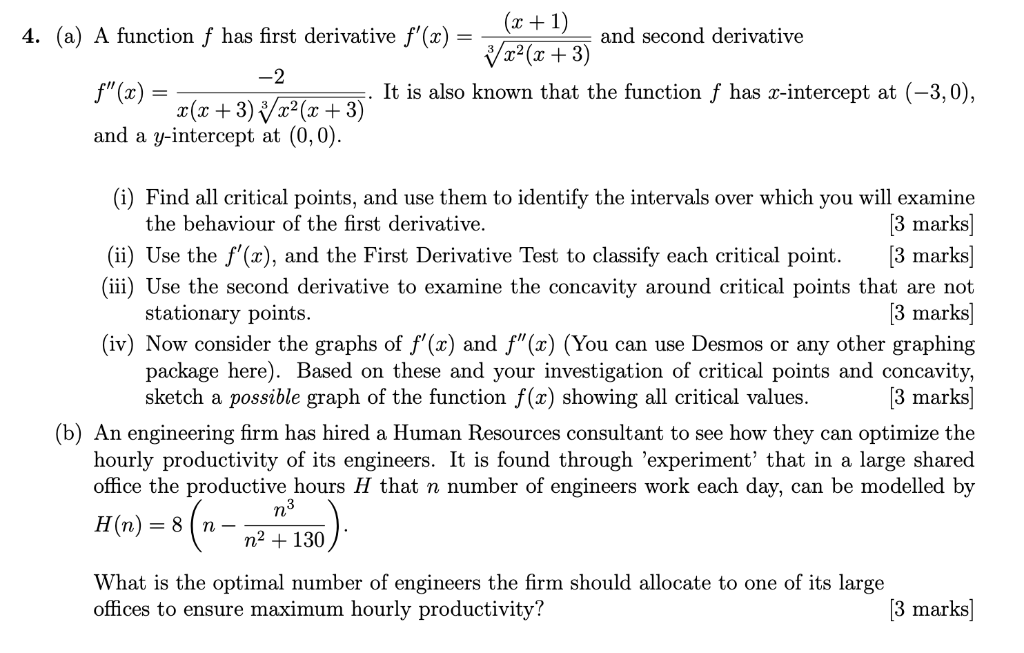4. (a) A function f has first derivative f'(x) and second derivative 2 f" (x) It is also known that the function f has r-intercept at (-3,0), and a y-intercept at (0,0) (i) Find all critical points, and use them to identify the intervals over which you will examine the behaviour of the first derivative. 3 marks] (ii) Use the f(x), and the First Derivative Test to classify each critical point.[3 marks] (iii) Use the second derivative to examine the...

• ### 4. (a) A function f has first derivative f'(r) and second derivative It is also known that the function f has r-int...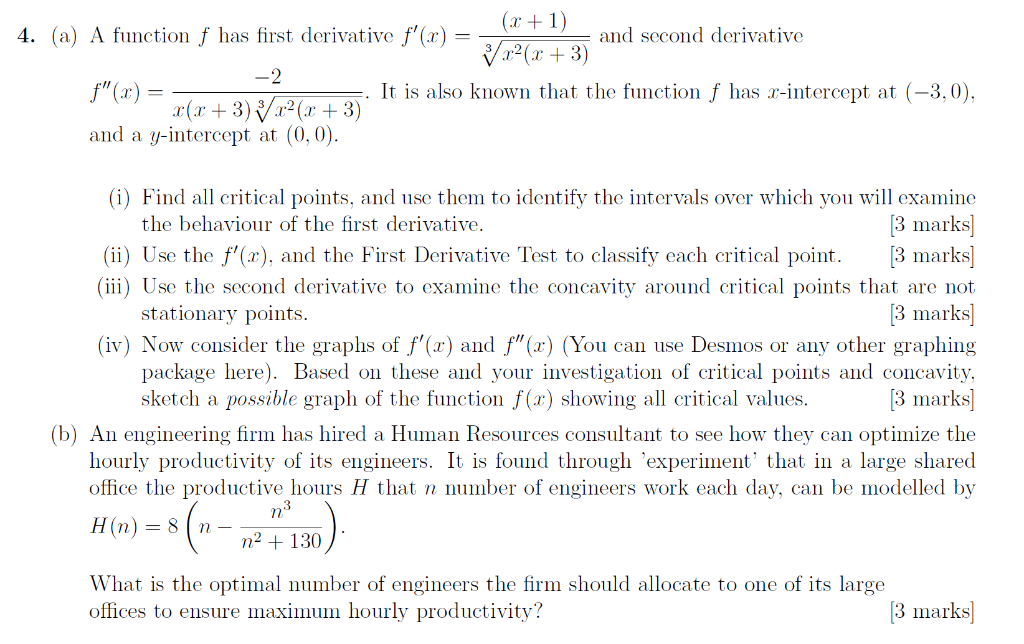4. (a) A function f has first derivative f'(r) and second derivative It is also known that the function f has r-intercept at (-3,0), and a y-intercept at (0, 0) i) Find all critical points, and use them to identify the intervals over which you will examine the behaviour of the first derivative 3 marks (İİ) Úse the f,(x), and the First Derivative Test to classify each critical point. [3 marks] Iİİ) Úse the second derivative to examine the concavity...

• ### I am having problems with this part of my assignment would i be able to get some help and show the working for these qu...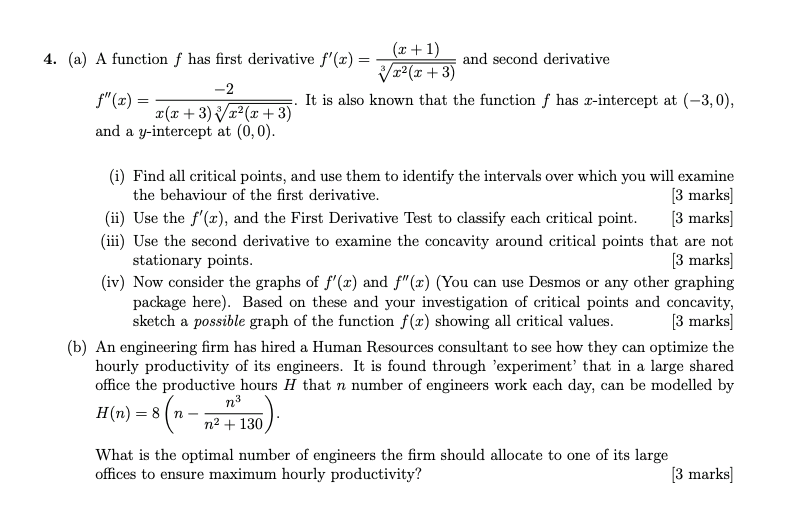I am having problems with this part of my assignment would i be able to get some help and show the working for these questions 4. (a) A function f has first derivative f'()- and second derivative 12 (z +3) f"(x) It is also known that the function f has r-intercept at (-3, 0), and a y-intercept at (0,0) (i) Find all critical points, and use them to identify the intervals over which you will examine the behaviour of the...

• ### 4. (a) A function f has first derivative f') and second derivative It is also known that the function f has r-i...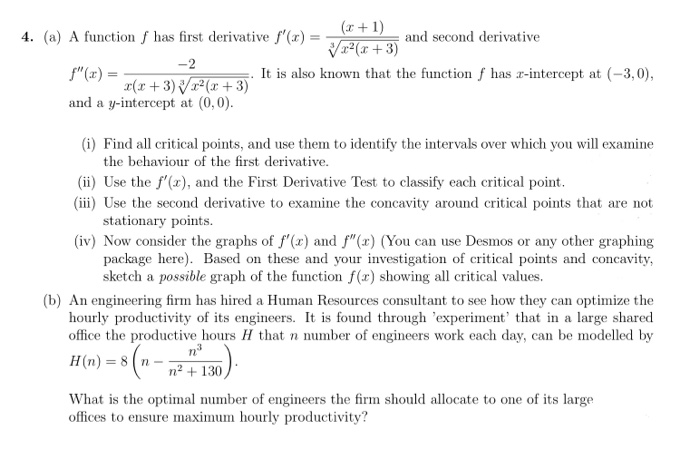4. (a) A function f has first derivative f') and second derivative It is also known that the function f has r-intercept at (-3,0) and a y-intercept at (0,0) 0) Find all critical points, and use them to identify the intervals over which you will examine the behaviour of the first derivative. (ii) Use the f'(x), and the First Derivative Test to classify each critical point. (ii) Use the second derivative to examine the concavity around critical points that are...

Need Online Homework Help?